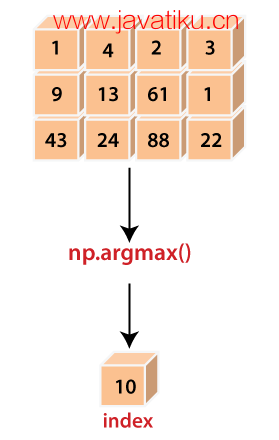# NumPy教程-numpy.argmax()在Python中的使用### 语法：

``numpy.argmax(a, axis=None, out=None)``

x: array_like

axis: int（可选）

out: array（可选）

### 示例 1：

``````Import numpy as np
x = np.arange(20).reshape(4,5) + 7
x
y=np.argmax(a)
y  ``````

``````array([[ 7,  8,  9, 10, 11],
[12, 13, 14, 15, 16],
[17, 18, 19, 20, 21],
[22, 23, 24, 25, 26]])
19``````

• 我们使用别名np导入了numpy模块。
• 我们使用np.arange()函数创建了一个数组'x'，形状为四行五列。
• 我们在每个元素中都加了7。
• 我们声明了变量'y'并将np.argmax()函数的返回值赋值给它。
• 我们在函数中传递了数组'x'
• 最后，我们尝试打印变量'y'

### 示例 2：

``````Import numpy as np
x = np.arange(20).reshape(4,5) + 7
y=np.argmax(x, axis=0)
z=np.argmax(x, axis=1)
y
z  ``````

``````array([3, 3, 3, 3, 3], dtype=int64)
array([4, 4, 4, 4], dtype=int64)``````

### 示例 3：

``````Import numpy as np
x = np.arange(20).reshape(4,5) + 7
indices = np.unravel_index(np.argmax(x, axis=None), x.shape)
indices
x[indices] ``````

``````(3, 4)
26``````

### 示例 4：

``````import numpy as np
a = np.array([[5,2,1], [3,7,9],[0, 4, 6]])
index_arr = np.argmax(a, axis=-1)
index_arr
# Same as np.max(a, axis=-1, keepdims=True)
result = np.take_along_axis(a, np.expand_dims(index_arr, axis=-1), axis=-1)
result1
# Same as np.max(a, axis=-1)
result = np.take_along_axis(a, np.expand_dims(index_arr, axis=-1), axis=-1).squeeze(axis=-1)
result2  ``````

``````array([,
,
])
array([5, 9, 6])``````

• 我们使用别名np导入了numpy模块。
• 我们使用np.array()函数创建了一个多维数组 'a' 。
• 我们声明了变量'index_arr'并将np.argmax()函数的返回值赋值给它。
• 我们在函数中传递了数组'a'和轴。
• 我们尝试打印变量'index_arr'
• 最后，我们尝试通过两种不同的方式获取数组中的最大值，这两种方式与np.argmax()相似。